Project tutorial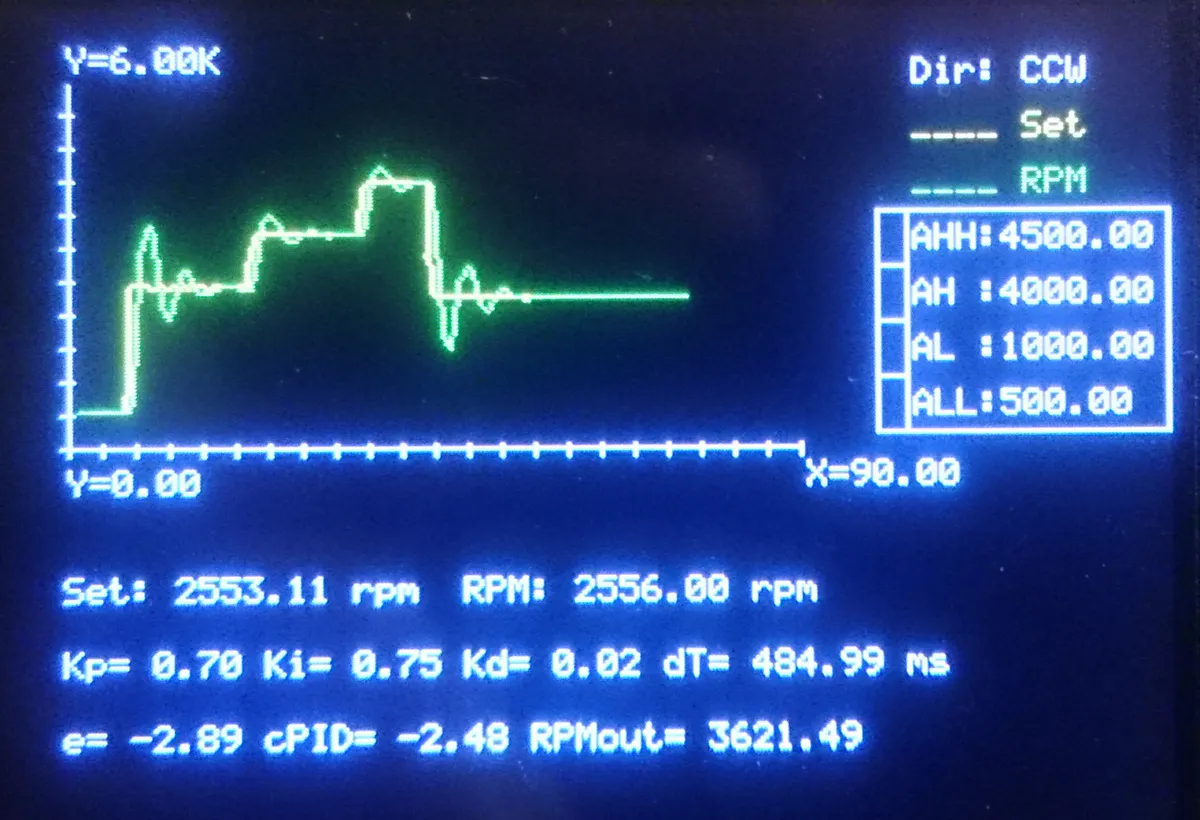Tricks for Controlling DC Motors © GPL3+

On this sample I would like to explain why PID-control should be used for speed controls and how the direction can be inverted.

• 33,287 views
• 94 respects

Components and supplies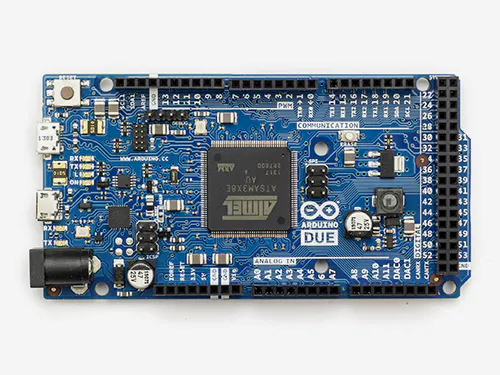Arduino Due Actually you can use any Arduino Board.
×1
 Makeblock Me TFT LCD This is an optional component. You can use another type of display, or prefer not to use any.
×1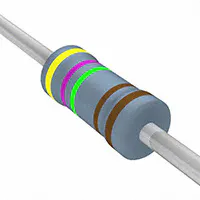Resistor 4.75k ohm Resistors may vary depending on your implementation.
×4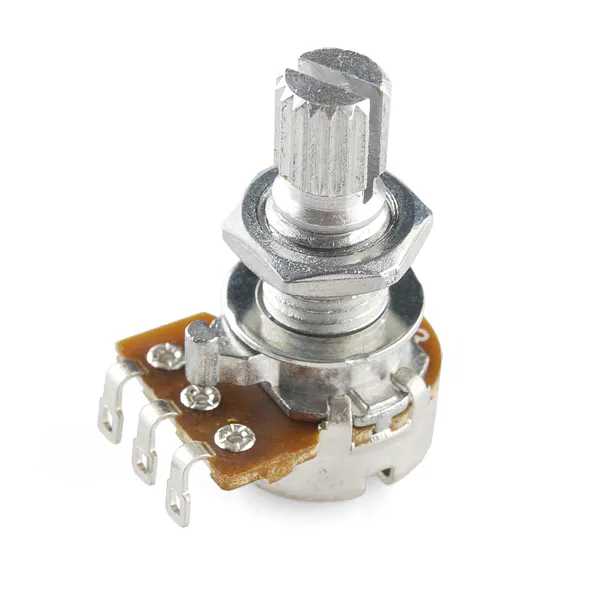Rotary potentiometer (generic) 3 of them are optional (just to adjust controller coefficients).
×4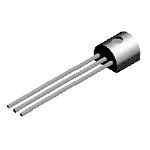General Purpose Transistor PNP Transistors to be used may vary depending on your implementation.
×4
 DC motor (generic)
×1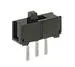Slide Switch This is used for direction selection.
×1
 Photoelectric Speed Sensor HC-020K
×1×1Jumper wires (generic)
×1

Apps and online services

Control of Speed and Direction of DC Motors by PID Controller and PWM Outputs

Intro

Almost on all available projects, originators would like to control motor speeds and direction together, but they prefer mainly directly sending PWM to DC motors, even via a motor control circuit. But such a method always fails if you need to match speed exactly as you desired because of twin brothers called as “friction” and “inertia”.

(Please never blame to Twins. Whatever and whenever you would like to take some actions with or without something, Twins immediately come and take action just to help you to keep everything under control. While Inertia lets things to “think” before taking action, Friction limits their acceleration and speed. And "power" is "nothing" if it's not under full control.)

So, if you try to control speed of a motor directly by sending your input as PWM signal to output, actual speed shall never meet with your set point and there will be significant difference (error), as can be seen on image just above. Here we need another way, and it’s called as “PID control”.

PID Controller

What’s PID control? Imagine how to drive your car: To start moving from full stop, you have to depress gas pedal more than during normal cruise. During moving at (almost) constant speed, you don’t need to depress gas pedal too much, but you just recover the loss of speed when needed. Besides, you release it slightly if acceleration is higher than your needs. This is also the way of "efficient driving".

So, PID controller does exactly the same: Controller reads difference “Error Signal (e)” between set point and actual output. It has 3 different components called as “Proportional”, “Integral” and “Derivative”; so controller’s name comes up after first letter of each one. Proportional component simply defines slope (acceleration) of controller output with respect to actual error signal. Integral part sums error signals in time in order to minimize final error. And Derivative component watches out the acceleration of error signal, and puts an “adjustment”. I will not give here further and longer details, please search on internet for further if you interest.

On my Arduino program, PID controller is written as a function shown below:

float controllerPID(float _E,  float _Eprev, float _dT,
float _Kp, float _Ki,    float _Kd)
{
float P, I, D;
/*
Base Formula:
U = _Kp * ( _E + 0.5*(1/_Ki)*(_E+_Eprev)*_dT + _Kd*(_E-_Eprev)/_dT );
*/
P = _Kp * _E; /* Proportional Component */
I = _Kp * 0.5 * _Ki * (_E+_Eprev) * _dT; /* Integral Component */
D = _Kp * _Kd * (_E-_Eprev) / _dT; /* Derivative Component */
return (P+I+D);
}

Then final output value is determined simply by adding current output value and output of PID controller. It’s the following section of main program together with calculation of Error Signal and PID Controller Output:

/* Error Signal, PID Controller Output and
Final Output (PWM) to Motor */
E = RPMset - RPM;
float cPID = controllerPID(E, Eprev, dT, Kp, Ki, Kd);
if ( RPMset == 0 ) OutputRPM = 0;
else OutputRPM = OutputRPM + cPID;
if ( OutputRPM < _minRPM ) OutputRPM = _minRPM;

DC Motor Supply Circuit

Of course it’s never recommended to drive a DC motor directly from output of Arduino or similar control board. DC motors need significant amount of current comparing to those cannot be supplied by outputs of controller cards. So you need to drive relay coils. But here another problem comes up: Relays have mechanical parts and they can fail in mid- or long term. We need another component here, transistors.

Actually DC motors are driven by current, not by voltage. So by using this principle, I decided to use transistors. But you have to pick up the correct transistor able to withstand motor current. At first, run motor directly by connecting to power supply, and measure current at the maximum operating conditions, or refer to manufacturer specifications.

After doing this, I decided to use four BC307A PNP bipolar junction transistors on a “bridge” to determine direction of current through motor coils (actually an NPN BC337 set would work better due to ability of withstanding significantly higher collector currents, but I didn't have them at the time).

Since motor currents should be passed through Emitter-Collector path of transistor, it’s necessary to use transistors with approximately same DC Current Gain (hfe) coefficients. To check it out, you can use following circuit, and collect transistors giving you approximately the same current reading on ampermeter. To design those preliminary circuits, you have consider followings:

• Find “Base-Emitter On-Voltage” (VBEon) of transistor. It’s the minimum voltage to be applied to Base for switching transistor on.
• Find typical “DC Current Gain” (hfe) of transistor at around the Collector Current close to Motor Current. Typically it’s the ratio between Collector Current (IC) and Base Current (IB), hfe = IC / IB.
• Find “Maximum Continuous Collector Current” of transistors (ICmax). The DC current of motor should never exceed this value in terms of absolute values. I can use BC307 since the motor I use needs on 70 mA while transistor has ICmax(abs) = 100 mA.

Now you can determine the resistor value to be connected to Base: At first, you have to consider limitations of your controller card's output, and try to keep Base current as minimum as possible (so it's recommended to select transistors' DC Current Gain as maximum as possible. Take the voltage rating of output on controller board as “Trigger Voltage” (VT), and find the Required Base Current (IBreq) by dividing Motor Current (IM) to DC Current Gain (hfe) of transistor: IBreq = IM / hfe.

Then determine the Voltage to be dropped across Resistor (VR), by subtracting Base-Emitter On-Voltage (VBEon) from Trigger Voltage: VR = VT - VBEon.

Finally divide Voltage to be dropped across Resistor (VR) to Required Base Current (IBreq) to find Resistor Value (R): R = VR / IBreq.

[ Combined formulation: R = ( VT - VBEon ) * hfe / IM ]

On my case:

• Motor Current: IM = 70 mA
• BC307A Parameters: ICmax = 100 mA, hfe = 140 (I measured approximately), VBEon = 0.62 V
• Trigger Voltage: VT = 3.3 V (PWM output of Arduino Due)
• R = 5360 ohm (So I decided to use 4900 ohms made by a 2K2 and 2K7, to ensure full RPM range coverage and circuit just sucks ~0.6 mA from PWM output - a suitable design.)

Reverting Direction and Important Notes

To revert direction of a DC motor, it’s enough to revert current flow. To do that, we can simply make a bridge circuit with four transistor sets. On the schematic; PWM Output#2 activates T1A and T1B while PWM Output#3 activates T2A and T2B, so the current passing through motor is changed.

But here we have to consider another issue: When just started, electric motors suck transient start-up current significantly higher than nominal current you read during normal/continuous operation (manufacturers give only nominal currents). Start-up current might be approximately 130% of nominal one for small power motors, and increases depending on motor power. So, if you feed motor directly from a voltage source and immediately reverse polarity during operation, motor sucks extreme levels of current since it’s not fully stopped. Finally this may result blowing out the power source or burning motor coils. This may not be so important and felt for very small power motors, but becomes important if power levels you’re working on increased. But if you're powering up motor through a transistor or a set of transistors (like Darlington Couple), you don't have such a problem since transistors already limit the current.

Anyway, I considered a small routine on program: When direction selection is changed during running, the program drives both command outputs to zero at first, and waits for motor until full stop. Then it finishes its duty, and gives all control back to the main routine.

if ( Direction != prevDirection )
{
/* Killing both of the PWM outputs to motor */
analogWrite(_chMotorCmdCCW,0); analogWrite(_chMotorCmdCW,0);
/* Wait until motor speed decreases */
do
while ( RPM > _minRPM );
}

On my application I used a cheap HC-020K speed sensor. It send pulses at the level of its supply voltage, and datasheet says that supply voltage is 5V. However my board is Arduino Due and it cannot accept it. So I directly powered it from Due’s 3.3V output, and yes, it worked. And following function is written to read frequency and HC-020K output.

{
pinMode(_DI_FrequencyCounter_Pin,INPUT);
unsigned long _Time = 0, _Time_Init;
float _Frequency = 0;
else
{
_Time_Init = micros();
do
{
_Time = micros();
}
while ( _Time < (_Time_Init + (1000000/_ReadingSpeed)) );
}
}

Note that HC-020K’s wheel has 20 slots, simply read frequency is to be divided by 20 in order to get revolution per second as frequency. Then result should be multiplied by 60 to get RPM.

Graphical Touch-Ups

In order display the inputs and results, I used a Makeblock Me TFT LCD and wrote CommandToTFT() function to send commands to it. Actually reason of this function is just to change serial connection point just at a single row in program, when needed.

Cartesian_Setup(), Cartesian_ClearPlotAreas() and Cartesian_Line() functions are written to prepare graphic plotting area, clear plot area when reached to the end of horizontal axis (here it’s “time”) and plot graphics respectively. Refer to Makeblock Me TFT LCD manual for further details if you interest graphical functions here, because I will not explain them here since they're actually out of this blog's scope.

Ending

Here I copy the program with- and without graphical functions separately, so you can review Speed and Direction Control Implementation standalone, or with graphics. Besides, on the Codes you can find further explanations for programmed functions.

Finally, normally you cannot decrease speed of a DC motor below 10-20% of its rated speed, even there's no any load. However, it's possible to decrease down to nearly 5% by using PID-control for an unloaded DC-motor once it's started.

Edit (25.Feb.2018): If you would like to use NPN transistors instead of PNP, you have to consider also reverse current flow on both types. When triggered (switched on) current flows from Emitter to Collector on PNP transistors, but it's reverse for NPN types (from Collector to Emitter). Hence PNP transistors are polarized as E(+) C(-) and for NPN it should be C(+) E(-).

Code

Code with Graphical Touch-UpsArduino
/* ###############################################
Color constants for Makeblock Me TFT LCD
############################################### */
#define _BLACK  0
#define _RED    1
#define _GREEN  2
#define _BLUE   3
#define _YELLOW 4
#define _CYAN   5
#define _PINK   6
#define _WHITE  7

/* ###############################################
I/O Assignments
############################################### */
int _chSpeedSet    = A0,  // Speed setpoint
_chKp          = A1,  // Proportional coefficient reading for PID controller
_chKi          = A2,  // Integral coefficient reading for PID controller
_chKd          = A3,  // Derivative coefficient reading for PID controller
_chMotorCmdCCW = 3,   // PWM output to motor for counter-clockwise turn
_chMotorCmdCW  = 2,   // PWM output to motor for clockwise turn
_chDirection   = 25;  // Direction selector reading

/* ###############################################
Other Constants
############################################### */
#define _minRPM        0  // Minimum RPM to initiate direction changing
#define _maxRPM     6000  // Maximum RPM limit
#define _Tmax         90  // Maximum time limit for graphing
#define _DiscSlots    20  // Qty of slots on Index Disc

/* ###############################################
Global Variables
############################################### */
String  Cartesian_SetupDetails;
boolean Direction, prevDirection;
// Alarm Settings
float RALL=500.0, RAL=1000.0, RAH=4000.0, RAHH=4500.0;
float Seconds=0.0, prevSeconds=0.0,
prevRPM=0.0, prevRPMset=0.0,
RPM=0.0,     RPMset=0.0, OutputRPM=0.0,
Kp=0.0,    Ki=0.0,       Kd=0.0,
Kpmax=2.0, Kimax=1.0,    Kdmax=1.0,
E=0.0,     Eprev=0.0,    dT=1.0;

/* ###############################################
CommandToTFT(TFTCmd)
Command Function for Makeblock Me TFT LCD
Input Parameters:
(String) TFTCmd : Command string
############################################### */
void CommandToTFT(String TFTCmd)
{
/* Serial Connection used for display */
Serial1.println(TFTCmd); delay(5);
}
/* ########### End of CommandToTFT() ########### */
/* ############################################# */

/* ###############################################
Cartesian_Setup(Xmin, Xmax, Ymin, Ymax, Window_X1, Window_Y1, Window_X2, Window_Y2, MinDashQty, ColorF, ColorX, ColorY)
Cartesian X-Y Axis Drawing Function for Makeblock Me TFT LCD
Input Parameters:
(float) Xmin, Xmax, Ymin, Ymax : Axis range values
(int)   Window_X1, Window_Y1___: Upper-left corner of graph window
(int)   Window_X2, Window_Y2___: Lower-right corner of graph window
(int)   MinDashQty_____________: Qty.of dashes on shortest axis
(int)   ColorB, ColorX, ColorY : Drawing colors for Frame, X-axis and Y-axis
Uses external function CommandToTFT().
############################################### */
String Cartesian_Setup(
float Xmin, float Xmax, float Ymin, float Ymax,
int Window_X1, int Window_Y1, int Window_X2, int Window_Y2,
int MinDashQty, int ColorF, int ColorX, int ColorY
)
{
/* Screen Limitations */
const int DisplayResolutionX = 319, DisplayResolutionY = 239;
/* Limit Title Strings */
String XminTxt;
if (abs(Xmin)>=1000000000)        XminTxt = "X=" + String (Xmin/1000000000) + "G";
else if (abs(Xmin)>=1000000)   XminTxt = "X=" + String (Xmin/1000000) + "M";
else if (abs(Xmin)>=1000) XminTxt = "X=" + String (Xmin/1000) + "K";
else                 XminTxt = "X=" + String (Xmin);
String XmaxTxt;
if (abs(Xmax)>=1000000000)        XmaxTxt = "X=" + String (Xmax/1000000000) + "G";
else if (abs(Xmax)>=1000000)   XmaxTxt = "X=" + String (Xmax/1000000) + "M";
else if (abs(Xmax)>=1000) XmaxTxt = "X=" + String (Xmax/1000) + "K";
else                 XmaxTxt = "X=" + String (Xmax);
String YminTxt;
if (abs(Ymin)>=1000000000)        YminTxt = "Y=" + String (Ymin/1000000000) + "G";
else if (abs(Ymin)>=1000000)   YminTxt = "Y=" + String (Ymin/1000000) + "M";
else if (abs(Ymin)>=1000) YminTxt = "Y=" + String (Ymin/1000) + "K";
else                 YminTxt = "Y=" + String (Ymin);
String YmaxTxt;
if (abs(Ymax)>=1000000000)        YmaxTxt = "Y=" + String (Ymax/1000000000) + "G";
else if (abs(Ymax)>=1000000)   YmaxTxt = "Y=" + String (Ymax/1000000) + "M";
else if (abs(Ymax)>=1000) YmaxTxt = "Y=" + String (Ymax/1000) + "K";
else                 YmaxTxt = "Y=" + String (Ymax);
/* Limits */  int XminPx = Window_X1+1; int XmaxPx = Window_X2-1;
int YmaxPx = Window_Y1+1; int YminPx = Window_Y2-1;
/* Origin */  int OriginX = XminPx + (int)( (XmaxPx - XminPx) * abs(Xmin) / (abs(Xmax)+abs(Xmin)) );
int OriginY = YmaxPx + (int)( (YminPx - YmaxPx) * abs(Ymax) / (abs(Ymax)+abs(Ymin)) );
/* Frame */   CommandToTFT ( "BOX(" + String(Window_X1) + "," + String(Window_Y1)+ "," +
String(Window_X2) + "," + String(Window_Y2)+ "," +
String(ColorF)    + ");"
);
/* X Axis */  CommandToTFT ( "PL(" + String(Window_X1+1) + "," + String(OriginY) + "," +
String(Window_X2-1) + "," + String(OriginY) + "," +
String(ColorX)            + ");"
);
/* Y Axis */  CommandToTFT ( "PL(" + String(OriginX) + "," + String(Window_Y1+1) + "," +
String(OriginX) + "," + String(Window_Y2-1) + "," +
String(ColorY)            + ");"
);

/*
Dashing: Minimum amount of dashes is given by "MinDashQty" and will be dashed on the shortest
axis-side with respect to origin.
On the other sections, dashes to be marked shall be determined by considering ratio to
shortest axis-side.
*/
/* Dashing */  int XlengthLeft  = abs(XminPx-OriginX);   int XlengthRight = abs(XmaxPx-OriginX);
int YlengthLower = abs(YminPx-OriginY);   int YlengthUpper = abs(YmaxPx-OriginY);
int XlengthLeft_Mod, XlengthRight_Mod, YlengthLower_Mod, YlengthUpper_Mod;
if (XlengthLeft<=1)  XlengthLeft_Mod=32767;  else XlengthLeft_Mod=XlengthLeft;
if (XlengthRight<=1) XlengthRight_Mod=32767; else XlengthRight_Mod=XlengthRight;
if (YlengthLower<=1) YlengthLower_Mod=32767; else YlengthLower_Mod=YlengthLower;
if (YlengthUpper<=1) YlengthUpper_Mod=32767; else YlengthUpper_Mod=YlengthUpper;
int MinAxisLength = min ( min (XlengthLeft_Mod,XlengthRight_Mod), min (YlengthLower_Mod,YlengthUpper_Mod) );
int XdashesLeft  = MinDashQty * XlengthLeft / MinAxisLength;
int XdashesRight = MinDashQty * XlengthRight / MinAxisLength;
int YdashesLower = MinDashQty * YlengthLower / MinAxisLength;
int YdashesUpper = MinDashQty * YlengthUpper / MinAxisLength;
int DashingInterval=2; // Min.interval btw.dashes

/* X-Dash L */  DashingInterval  = (int) (XlengthLeft / XdashesLeft);
if (!(DashingInterval<2))
for (int i=OriginX; i>=XminPx; i-=DashingInterval)
CommandToTFT ( "PL(" + String(i) + "," + String(OriginY-2) + "," +
String(i) + "," + String(OriginY+2) + "," +
String(ColorX)  + ");"
);
/* X-Dash R */  DashingInterval  = (int) (XlengthRight / XdashesRight);
if (!(DashingInterval<2))
for (int i=OriginX; i<=XmaxPx; i+=DashingInterval)
CommandToTFT ( "PL(" + String(i) + "," + String(OriginY-2) + "," +
String(i) + "," + String(OriginY+2) + "," +
String(ColorX)  + ");"
);
/* Y-Dash-L */  DashingInterval  = (int) (YlengthLower / YdashesLower);
if (!(DashingInterval<2))
for (int i=OriginY; i<=YminPx; i+=DashingInterval)
CommandToTFT ( "PL(" + String(OriginX-2) + "," + String(i) + "," +
String(OriginX+2) + "," + String(i) + "," +
String(ColorY)  + ");"
);
/* Y-Dash-U */  DashingInterval  = (int) (YlengthUpper / YdashesUpper);
if (!(DashingInterval<2))
for (int i=OriginY; i>=YmaxPx; i-=DashingInterval)
CommandToTFT ( "PL(" + String(OriginX-2) + "," + String(i) + "," +
String(OriginX+2) + "," + String(i) + "," +
String(ColorY)  + ");"
);

/* Calculating coordinates to display axis endpoint values */
int XminTxtX = Window_X1 - (int)(XminTxt.length()*6) - 1,
XminTxtY = OriginY,
XmaxTxtX = Window_X2 + 1,
XmaxTxtY = OriginY,
YminTxtX = OriginX,
YminTxtY = Window_Y2 + 1,
YmaxTxtX = OriginX,
YmaxTxtY = Window_Y1 - 12 - 1;
/*  Controls: If any coordinate is -1, it shall fall beyond display limits
and respective value shall not be displayed */
if (XminTxtX<0) XminTxtX = -1;
if ( (XminTxtY-12) < 0 ) XminTxtY = -1;
if ( (XmaxTxtX+6*XmaxTxt.length()) > DisplayResolutionX ) XmaxTxtX = -1;
if ( (XmaxTxtY+12) > DisplayResolutionY ) XmaxTxtY = -1;
if ( (YminTxtX+6*YminTxt.length()) > DisplayResolutionX ) YminTxtX = -1;
if ( (YminTxtY+12) > DisplayResolutionY ) YminTxtY = -1;
if ( (YmaxTxtX+6*YmaxTxt.length()) > DisplayResolutionX ) YmaxTxtX = -1;
if (YmaxTxtY<0) YmaxTxtY = -1;

/* Range Limit Titles */
if ( ( XminTxtX != -1 ) && ( XminTxtY != -1 ) )
CommandToTFT( "DS12(" + String(XminTxtX) + "," + String(XminTxtY) + ",'" + String(XminTxt) + "'," + String(ColorX) + ");" );
if ( ( XmaxTxtX != -1 ) && ( XmaxTxtY != -1 ) )
CommandToTFT( "DS12(" + String(XmaxTxtX) + "," + String(XmaxTxtY) + ",'" + String(XmaxTxt) + "'," + String(ColorX) + ");" );
if ( ( YminTxtX != -1 ) && ( YminTxtY != -1 ) )
CommandToTFT( "DS12(" + String(YminTxtX) + "," + String(YminTxtY) + ",'" + String(YminTxt) + "'," + String(ColorY) + ");" );
if ( ( YmaxTxtX != -1 ) && ( YmaxTxtY != -1 ) )
CommandToTFT( "DS12(" + String(YmaxTxtX) + "," + String(YmaxTxtY) + ",'" + String(YmaxTxt) + "'," + String(ColorY) + ");" );

/*
Return Value String
Cartesian_Setup() will return a string packing graphic configuration in following format:
"<Xmin,Xmax,Ymin,Ymax,Window_X1,Window_Y1,Window_X2,Window_Y2>"
String starts with '<' and ends by '>'. Each value is delimited by ','
*/
/* Initialize    */ String Cartesian_SetupDetails = "<";
Cartesian_SetupDetails += ( String(Xmin) + "," );
Cartesian_SetupDetails += ( String(Xmax) + "," );
Cartesian_SetupDetails += ( String(Ymin) + "," );
Cartesian_SetupDetails += ( String(Ymax) + "," );
Cartesian_SetupDetails += ( String(Window_X1) + "," );
Cartesian_SetupDetails += ( String(Window_Y1) + "," );
Cartesian_SetupDetails += ( String(Window_X2) + "," );
Cartesian_SetupDetails += ( String(Window_Y2) + "," );
/* Close-Out     */ Cartesian_SetupDetails += ">";

return Cartesian_SetupDetails;
}
/* ########### End of Cartesian_Setup() ########### */
/* ################################################ */

/* ###############################################
Cartesian_ClearPlotAreas(Descriptor, Color)
Plot Area Reset/Clear Function for Makeblock Me TFT LCD
Input Parameters:
(String) Descriptor : Setup Descriptor - returned by Cartesian_Setup()
(int)    Color______: Color to be used to fill plot area
Uses external function CommandToTFT().
############################################### */
void Cartesian_ClearPlotAreas(String Descriptor, int Color)
{
int X1,Y1,X2,Y2;      /* Boundary coordinates for plot areas */
/* Extracting values from Descriptor */
/* L L L L W      W      W      W       */
/* Xmin Xmax Ymin Ymax Window_X1 Window_Y1 Window_X2 Window_Y2  */
float L; int W; /* Values stored in Descriptor */
int j=0;              /* Counter */
String D_Str = "";
for (int i=1; i<=(Descriptor.length()-1); i++)
if ( Descriptor[i] == ',' )
{
if (j<4) L[j]=D_Str.toFloat(); else W[j-4]=D_Str.toInt();
D_Str=""; j++;
}
else
D_Str += Descriptor[i];

/* Origin */  int OriginX = (W+1) + (int)( ( (W-1) - (W+1) ) * abs(L) / (abs(L)+abs(L)) );
int OriginY = (W+1) + (int)( ( (W-1) - (W+1) ) * abs(L) / (abs(L)+abs(L)) );

/* Clearing Plot Areas */
//Area.1 : X+ Y+
X1 = OriginX + 2 ; Y1 = W + 1 ;
X2 = W - 1    ; Y2 = OriginY - 2 ;
CommandToTFT ( "BOXF(" + String(X1) + "," + String(Y1) + "," +
String(X2) + "," + String(Y2) + "," +
String(Color)     + ");"
);
//Area.2 : X- Y+
X1 = W + 1 ;    Y1 = W + 1 ;
X2 = OriginX - 2 ; Y2 = OriginY - 2 ;
CommandToTFT ( "BOXF(" + String(X1) + "," + String(Y1) + "," +
String(X2) + "," + String(Y2) + "," +
String(Color)     + ");"
);
//Area.3 : X- Y-
X1 = W + 1 ;    Y1 = OriginY + 2 ;
X2 = OriginX - 2 ; Y2 = W - 1 ;
CommandToTFT ( "BOXF(" + String(X1) + "," + String(Y1) + "," +
String(X2) + "," + String(Y2) + "," +
String(Color)     + ");"
);
//Area.4 : X+ Y-
X1 = OriginX + 2 ; Y1 = OriginY + 2 ;
X2 = W - 1 ;    Y2 = W - 1 ;
CommandToTFT ( "BOXF(" + String(X1) + "," + String(Y1) + "," +
String(X2) + "," + String(Y2) + "," +
String(Color)     + ");"
);
}
/* ########### End of Cartesian_ClearPlotAreas() ########### */
/* ######################################################### */

/* ###############################################
Cartesian_Line(Xp, Yp, X, Y, Descriptor, Color)
Cartesian Line Function for Makeblock Me TFT LCD
Input Parameters:
(int)    Xp, Yp_____: Previous plot coordinates - y value vs x
(int)    X, Y_______: Current plot coordinates - y value vs x
(String) Descriptor : Setup Descriptor - returned by Cartesian_Setup()
(int)    Color______: Marking color to be used on (x,y)
Uses external function CommandToTFT().
############################################### */
void Cartesian_Line(float Xp, float Yp, float X, float Y, String Descriptor, int Color)
{
/* Extracting values from Descriptor */
/* L L L L W      W      W      W       */
/* Xmin Xmax Ymin Ymax Window_X1 Window_Y1 Window_X2 Window_Y2  */
float L; int W; /* Values stored in Descriptor */
int j=0;              /* Counter */
String D_Str = "";
for (int i=1; i<=(Descriptor.length()-1); i++)
if ( Descriptor[i] == ',' )
{
if (j<4) L[j]=D_Str.toFloat(); else W[j-4]=D_Str.toInt();
D_Str=""; j++;
}
else
D_Str += Descriptor[i];

/* Origin */  int OriginX = (W+1) + (int)( ( (W-1) - (W+1) ) * abs(L) / (abs(L)+abs(L)) );
int OriginY = (W+1) + (int)( ( (W-1) - (W+1) ) * abs(L) / (abs(L)+abs(L)) );

int XminPx = W + 1;
int XmaxPx = W - 1;
int YmaxPx = W + 1;
int YminPx = W - 1;

if (Y>L) Y=L;
if (Y<L) Y=L;

float RatioX = (float)( XmaxPx - XminPx ) / ( L - L );
float RatioY = (float)abs( YmaxPx - YminPx ) / ( L - L );

int DispXp = XminPx + (int)( RatioX * ( Xp - L ) );
int DispYp = YminPx - (int)( RatioY * ( Yp - L ) );
int DispX = XminPx + (int)( RatioX * ( X - L ) );
int DispY = YminPx - (int)( RatioY * ( Y - L ) );
if (!(
( ( DispXp >= (OriginX-2) ) && ( DispXp <= (OriginX+2) ) ) ||
( ( DispYp >= (OriginY-2) ) && ( DispYp <= (OriginY+2) ) ) ||
( ( DispX >= (OriginX-2) ) && ( DispX <= (OriginX+2) ) ) ||
( ( DispY >= (OriginY-2) ) && ( DispY <= (OriginY+2) ) )
))
CommandToTFT( "PL(" + String(DispXp) + "," + String(DispYp) + "," + String(DispX) + "," + String(DispY) + "," + String(Color) + ");" );
}
/* ########### End of Cartesian_Line() ########### */
/* ############################################### */

/* ###############################################
Input Parameters:
(int)   _DI_FrequencyCounter_Pin : Digital pin to be read

Note.1: _ReadingSpeed is a value to specify how long shall the changes be counted.
It cannot be 0(zero), negative values or a value greater than 10.
When _ReadingSpeed changed, 1 second shall be divided by this value to calculate
required counting duration. For example;
- _ReadingSpeed = 0.1 -> input shall be counted during 10 seconds (=1/0.1)
- _ReadingSpeed = 0.5 -> input shall be counted during 2 seconds (=1/0.5)
- _ReadingSpeed = 2.0 -> input shall be counted during 0.5 seconds (=1/2)
- _ReadingSpeed = 4.0 -> input shall be counted during 0.25 seconds (=1/4)
on lower frequencies (generally below 100 Hz) since counting error increases
up to 20%~40% by decreasing frequency.
############################################### */

{
pinMode(_DI_FrequencyCounter_Pin,INPUT);
unsigned long _Time = 0, _Time_Init;
float _Frequency = 0;
else
{
_Time_Init = micros();
do
{
_Time = micros();
}
while ( _Time < (_Time_Init + (1000000/_ReadingSpeed)) );
}
}
/* ########### End of readFrequency() ########### */
/* ############################################## */

/* ###############################################
controllerPID(RangeMin, RangeMax, _E, _Eprev, _dT, _Kp, _Ki, _Kd)
PID Controller Function
Input Parameters:
(float) RangeMin: Minimum limit for output
(float) RangeMax: Maximum limit for output
(float) _E_____: Current error signal
(float) _Eprev : Previous error signal
(float) _dT____: Time difference as seconds
(float) _Kp____: Proportional coefficient
(float) _Ki____: Integral coefficient
(float) _Kp____: Derivative coefficient
1. Set Kp=0, Ki=0, Kd=0.
2. Start to increase Kp until the system oscillates at fixed period (Pc) and note
critical gain Kc = Kp.
3. Adjust final coefficients as follows.
for P-control only  : Kp = 0.50*Kc
for PI-control only : Kp = 0.45*Kc, Ki = 1.2/Pc
for PID-control     : Kp = 0.60*Kc, Ki = 2.0/Pc, Kd=Pc/8
4. Fine tuning could be done by slightly changing each coefficient.
############################################### */

float controllerPID(float _E, float _Eprev, float _dT, float _Kp, float _Ki, float _Kd)
{
float P, I, D;
/*
Base Formula: U = _Kp * ( _E + 0.5*(1/_Ki)*(_E+_Eprev)*_dT + _Kd*(_E-_Eprev)/_dT );
*/
P = _Kp * _E;                             /* Proportional Component */
I = _Kp * 0.5 * _Ki * (_E+_Eprev) * _dT;  /* Integral Component */
D = _Kp * _Kd * (_E-_Eprev) / _dT;        /* Derivative Component */
return (P+I+D);
}
/* ########### End of controllerPID() ########### */
/* ############################################## */

/* ###############################################
Setup
############################################### */

void setup()
{
Serial1.begin(9600);
Serial1.println("CLS(0);");delay(20);

pinMode(_chMotorCmdCCW,OUTPUT); // PWM output to motor for counter-clockwise turn
pinMode(_chMotorCmdCW,OUTPUT);  // PWM output to motor for clockwise turn

// Initial killing the PWM outputs to motor
analogWrite(_chMotorCmdCCW,0); analogWrite(_chMotorCmdCW,0);

// Initial reading for direction selection
prevDirection=Direction;

// The section below prepares TFT LCD
// Cartesian_Setup(Xmin, Xmax, Ymin, Ymax, Window_X1, Window_Y1, Window_X2, Window_Y2, MinDashQty, ColorF, ColorX, ColorY)
Cartesian_SetupDetails = Cartesian_Setup(0, _Tmax, _minRPM, _maxRPM, 20, 20, 220, 120, 10, 0, 7, 7);
CommandToTFT("DS12(250,10,'Dir: CW '," + String(_WHITE) + ");");
CommandToTFT("DS12(250,25,'____ Set'," + String(_YELLOW) + ");");
CommandToTFT("DS12(250,40,'____ RPM'," + String(_GREEN) + ");");
/* Alarm Values */
CommandToTFT("DS12(250,55,'AHH:" + String(RAHH) + "'," + String(_WHITE) + ");");
CommandToTFT("DS12(250,70,'AH :" + String(RAH)  + "'," + String(_WHITE) + ");");
CommandToTFT("DS12(250,85,'AL :" + String(RAL)  + "'," + String(_WHITE) + ");");
CommandToTFT("DS12(250,100,'ALL:"+ String(RALL) + "'," + String(_WHITE) + ");");
/* Alarm Window */
CommandToTFT("BOX(240,55,319,115," + String(_WHITE) + ");");
/* Alarm Lamps */
CommandToTFT("BOX(240,55,248,70," + String(_WHITE) + ");");
CommandToTFT("BOX(240,70,248,85," + String(_WHITE) + ");");
CommandToTFT("BOX(240,85,248,100," + String(_WHITE) + ");");
CommandToTFT("BOX(240,100,248,115," + String(_WHITE) + ");");
}

/* ###############################################
Loop
############################################### */

void loop()
{

// Initialization Time: Necessary for PID controller.
int InitTime = micros();

// X-Axis Auto-Reset for Graphing
if ( Seconds > 90.0 )
{
Seconds = 0.0;
Cartesian_ClearPlotAreas(Cartesian_SetupDetails,0);
}

/* Controller Coefficients */
Kp = Kpmax * (float)analogRead(_chKp) / 4095;
Ki = Kimax * (float)analogRead(_chKi) / 4095;
Kd = Kdmax * (float)analogRead(_chKd) / 4095;
/* Direction Selector */
Direction = digitalRead(_chDirection);  /* HIGH=CCW, LOW=CW */
/* Actual RPM and RPM Setpoint
Note that maximum selectable RPM is 5000. */
RPMset = 5000 * (float)analogRead(_chSpeedSet) / 4095;

// Calculations and Actions
/* Error Signal, PID Controller Output and Final Output (PWM) to Motor */
E = RPMset - RPM;
float cPID = controllerPID(E, Eprev, dT, Kp, Ki, Kd);
if ( RPMset == 0 ) OutputRPM = 0;
else OutputRPM = OutputRPM + cPID;
if ( OutputRPM < _minRPM ) OutputRPM = _minRPM;
if ( OutputRPM > _maxRPM ) OutputRPM = _maxRPM;

/* Changing Direction when inverted
Note that no any graphical indication is performed on this function.  */
if ( Direction != prevDirection )
{
/* Killing both of the PWM outputs to motor */
analogWrite(_chMotorCmdCCW,0); analogWrite(_chMotorCmdCW,0);
/* Wait until motor speed decreases */
do
while ( RPM > _minRPM );
}

// Writing Outputs
if (Direction==HIGH) analogWrite(_chMotorCmdCCW,(int)(255*OutputRPM/_maxRPM));
else analogWrite(_chMotorCmdCW, (int)(255*OutputRPM/_maxRPM));

// Graphing
/* Indicating Direction */
if (Direction==HIGH) CommandToTFT("DS12(280,10,'CCW '," + String(_WHITE) + ");");
else CommandToTFT("DS12(280,10,'CW  '," + String(_WHITE) + ");");
/* Plotting Curve */
Cartesian_Line(prevSeconds, prevRPMset, Seconds, RPMset, Cartesian_SetupDetails, _YELLOW);
Cartesian_Line(prevSeconds, prevRPM, Seconds, RPM, Cartesian_SetupDetails, _GREEN);
/* Indicating values of RPM Setpoint, PID Controller Coefficients,
Error Signal, PID Controller Output and Final RPM Output (PWM) */
CommandToTFT( "DS12(20,150,'Set: " + String(RPMset) + " rpm  " +
"RPM: " + String(RPM)    + " rpm  '," + String(_WHITE) + ");");
CommandToTFT( "DS12(20,170,'Kp= " + String(Kp) + " " +
"Ki= " + String(Ki) + " " +
"Kd= " + String(Kd) + " " +
"dT= " + String(dT*1000) + " ms   '," + String(_WHITE) + ");");
CommandToTFT( "DS12(20,190,'e= "      + String(E)         + " " +
"cPID= "   + String(cPID)      + " " +
"RPMout= " + String(OutputRPM) + "         '," + String(_WHITE) + ");");
/* Resetting Alarm Lamps */
CommandToTFT("BOXF(241,56,247,69," + String(_BLACK) + ");");
CommandToTFT("BOXF(241,71,247,84," + String(_BLACK) + ");");
CommandToTFT("BOXF(241,86,247,99," + String(_BLACK) + ");");
CommandToTFT("BOXF(241,101,247,114," + String(_BLACK) + ");");
/* Activating Necessary Alarm Lamps */
if (RPM>=RAHH)              CommandToTFT("BOXF(241,56,247,69," + String(_RED) + ");");
if ((RPM>=RAH)&&(RPM<RAHH)) CommandToTFT("BOXF(241,71,247,84," + String(_RED) + ");");
if ((RPM>RALL)&&(RPM<=RAL)) CommandToTFT("BOXF(241,86,247,99," + String(_RED) + ");");
if (RPM<=RALL)              CommandToTFT("BOXF(241,101,247,114," + String(_RED) + ");");

// Storing Values generated on previous cycle
Eprev = E; prevRPMset = RPMset;   prevRPM = RPM;
prevSeconds = Seconds; prevDirection = Direction;

// Calculating control application cycle time and passed Seconds
dT = float ( micros() - InitTime ) / 1000000.0;
Seconds+=dT;
}
Code without Graphical Touch-UpsArduino
/* ###############################################
I/O Assignments
############################################### */
int _chSpeedSet    = A0,  // Speed setpoint
_chKp          = A1,  // Proportional coefficient reading for PID controller
_chKi          = A2,  // Integral coefficient reading for PID controller
_chKd          = A3,  // Derivative coefficient reading for PID controller
_chMotorCmdCCW = 3,   // PWM output to motor for counter-clockwise turn
_chMotorCmdCW  = 2,   // PWM output to motor for clockwise turn
_chDirection   = 25;  // Direction selector reading

/* ###############################################
Other Constants
############################################### */
#define _minRPM        0  // Minimum RPM to initiate direction changing
#define _maxRPM     6000  // Maximum RPM limit
#define _DiscSlots    20  // Qty of slots on Index Disc

/* ###############################################
Global Variables
############################################### */
boolean Direction, prevDirection;
float RPM=0.0,     RPMset=0.0, OutputRPM=0.0,
Kp=0.0,    Ki=0.0,       Kd=0.0,
Kpmax=2.0, Kimax=1.0,    Kdmax=1.0,
E=0.0,     Eprev=0.0,    dT=1.0;

/* ###############################################
Input Parameters:
(int)   _DI_FrequencyCounter_Pin : Digital pin to be read

Note.1: _ReadingSpeed is a value to specify how long shall the changes be counted.
It cannot be 0(zero), negative values or a value greater than 10.
When _ReadingSpeed changed, 1 second shall be divided by this value to calculate
required counting duration. For example;
- _ReadingSpeed = 0.1 -> input shall be counted during 10 seconds (=1/0.1)
- _ReadingSpeed = 0.5 -> input shall be counted during 2 seconds (=1/0.5)
- _ReadingSpeed = 2.0 -> input shall be counted during 0.5 seconds (=1/2)
- _ReadingSpeed = 4.0 -> input shall be counted during 0.25 seconds (=1/4)
on lower frequencies (generally below 100 Hz) since counting error increases
up to 20%~40% by decreasing frequency.
############################################### */

{
pinMode(_DI_FrequencyCounter_Pin,INPUT);
unsigned long _Time = 0, _Time_Init;
float _Frequency = 0;
else
{
_Time_Init = micros();
do
{
_Time = micros();
}
while ( _Time < (_Time_Init + (1000000/_ReadingSpeed)) );
}
}
/* ########### End of readFrequency() ########### */
/* ############################################## */

/* ###############################################
controllerPID(RangeMin, RangeMax, _E, _Eprev, _dT, _Kp, _Ki, _Kd)
PID Controller Function
Input Parameters:
(float) RangeMin: Minimum limit for output
(float) RangeMax: Maximum limit for output
(float) _E_____: Current error signal
(float) _Eprev : Previous error signal
(float) _dT____: Time difference as seconds
(float) _Kp____: Proportional coefficient
(float) _Ki____: Integral coefficient
(float) _Kp____: Derivative coefficient
1. Set Kp=0, Ki=0, Kd=0.
2. Start to increase Kp until the system oscillates at fixed period (Pc) and note
critical gain Kc = Kp.
3. Adjust final coefficients as follows.
for P-control only  : Kp = 0.50*Kc
for PI-control only : Kp = 0.45*Kc, Ki = 1.2/Pc
for PID-control     : Kp = 0.60*Kc, Ki = 2.0/Pc, Kd=Pc/8
4. Fine tuning could be done by slightly changing each coefficient.
############################################### */

float controllerPID(float _E, float _Eprev, float _dT, float _Kp, float _Ki, float _Kd)
{
float P, I, D;
/*
Base Formula: U = _Kp * ( _E + 0.5*(1/_Ki)*(_E+_Eprev)*_dT + _Kd*(_E-_Eprev)/_dT );
*/
P = _Kp * _E;                             /* Proportional Component */
I = _Kp * 0.5 * _Ki * (_E+_Eprev) * _dT;  /* Integral Component */
D = _Kp * _Kd * (_E-_Eprev) / _dT;        /* Derivative Component */
return (P+I+D);
}
/* ########### End of controllerPID() ########### */
/* ############################################## */

/* ###############################################
Setup
############################################### */

void setup()
{
pinMode(_chMotorCmdCCW,OUTPUT); // PWM output to motor for counter-clockwise turn
pinMode(_chMotorCmdCW,OUTPUT);  // PWM output to motor for clockwise turn
// Initial killing the PWM outputs to motor
analogWrite(_chMotorCmdCCW,0); analogWrite(_chMotorCmdCW,0);
// Initial reading for direction selection
prevDirection=Direction;
}

/* ###############################################
Loop
############################################### */

void loop()
{
// Initialization Time: Necessary for PID controller.
int InitTime = micros();

/* Controller Coefficients */
Kp = Kpmax * (float)analogRead(_chKp) / 4095;
Ki = Kimax * (float)analogRead(_chKi) / 4095;
Kd = Kdmax * (float)analogRead(_chKd) / 4095;
/* Direction Selector */
Direction = digitalRead(_chDirection);  /* HIGH=CCW, LOW=CW */
/* Actual RPM and RPM Setpoint
Note that maximum selectable RPM is 5000. */
RPMset = 5000 * (float)analogRead(_chSpeedSet) / 4095;

// Calculations and Actions
/* Error Signal, PID Controller Output and Final Output (PWM) to Motor */
E = RPMset - RPM;
float cPID = controllerPID(E, Eprev, dT, Kp, Ki, Kd);
if ( RPMset == 0 ) OutputRPM = 0;
else OutputRPM = OutputRPM + cPID;
if ( OutputRPM < _minRPM ) OutputRPM = _minRPM;
if ( OutputRPM > _maxRPM ) OutputRPM = _maxRPM;

/* Changing Direction when inverted */
if ( Direction != prevDirection )
{
/* Killing both of the PWM outputs to motor */
analogWrite(_chMotorCmdCCW,0); analogWrite(_chMotorCmdCW,0);
/* Wait until motor speed decreases */
do
while ( RPM > _minRPM );
}

// Writing Outputs
if (Direction==HIGH) analogWrite(_chMotorCmdCCW,(int)(255*OutputRPM/_maxRPM));
else analogWrite(_chMotorCmdCW, (int)(255*OutputRPM/_maxRPM));

// Storing Values generated on previous cycle
Eprev = E; prevDirection = Direction;

// Calculating control application cycle time and passed Seconds
dT = float ( micros() - InitTime ) / 1000000.0;
}

Schematics

It's a prototype to explain DC motor speed control by using PID controller, and what should be considered for reversing.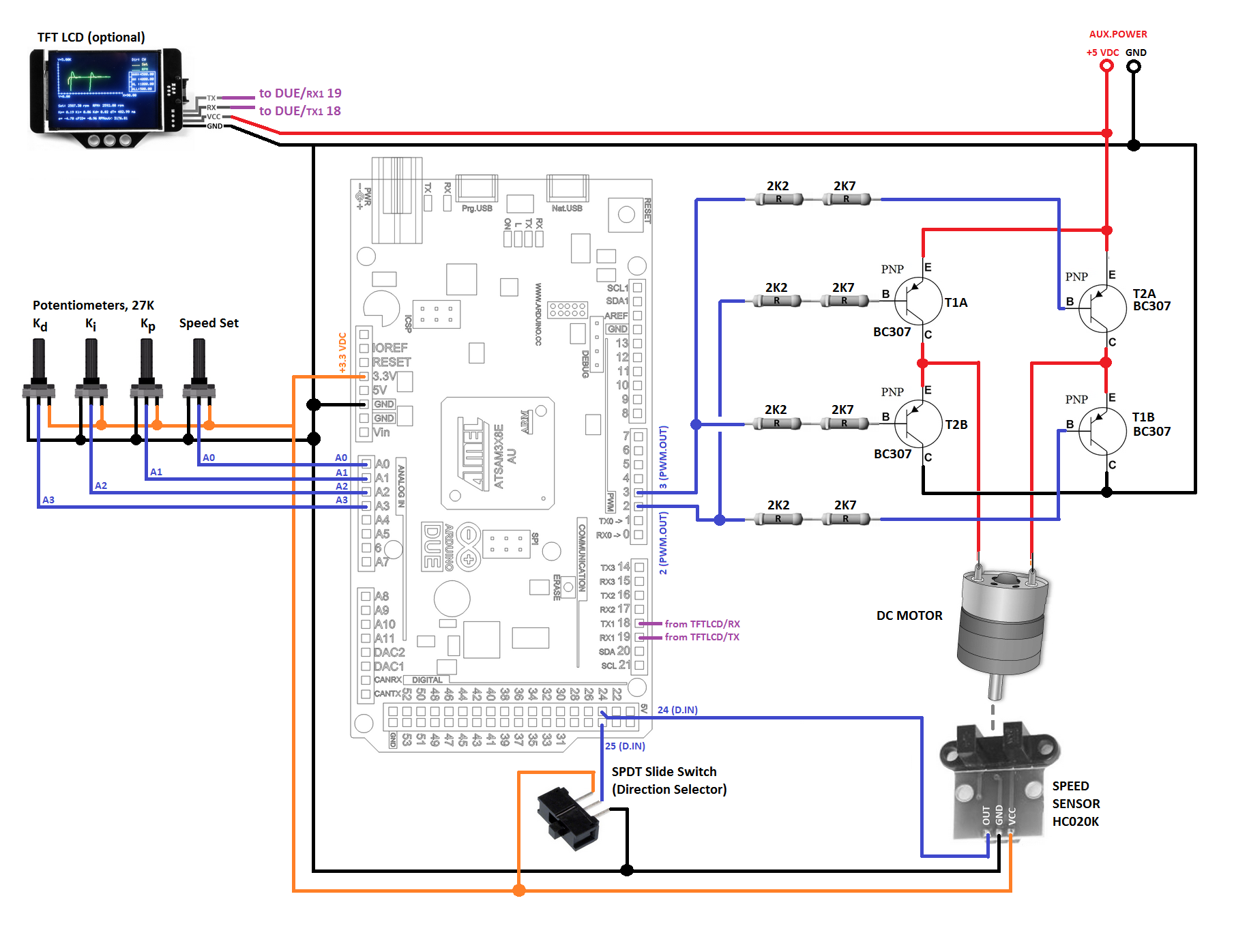DC Motor Controlling Library

Project tutorial by ambhatt

• 46,720 views
• 61 respects

Solar Tracker 35W with DC Motors

Project showcase by DemetrisEng

• 31,150 views
• 71 respects

Controlling Appliances Using Android Device and Arduino

Project tutorial by Siddhartha mudgal

• 4,995 views
• 11 respects

Nano IR Remote for DC Motors

Project showcase by Boaz Lawnce

• 4,230 views
• 7 respects

Control the Speed of Brushless DC Motor Using Bluetooth

Project tutorial by mohitmehlawat

• 15,039 views